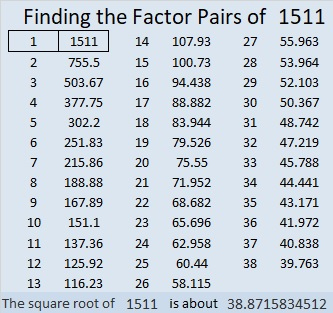# 1511 and Level 1

Contents

### Today’s Puzzle:

Do you know what number is the common factor of all the clues in this puzzle? If you do, then you are well on your way to solving this level one puzzle!### Factors of 1511:

• 1511 is a prime number.
• Prime factorization: 1511 is prime.
• 1511 has no exponents greater than 1 in its prime factorization, so √1511 cannot be simplified.
• The exponent in the prime factorization is 1. Adding one to that exponent we get (1 + 1) = 2. Therefore 1511 has exactly 2 factors.
• The factors of 1511 are outlined with their factor pair partners in the graphic below.How do we know that 1511 is a prime number? If 1511 were not a prime number, then it would be divisible by at least one prime number less than or equal to √1511. Since 1511 cannot be divided evenly by 2, 3, 5, 7, 11, 13, 17, 19, 23, 29 31, or 37, we know that 1511 is a prime number.

### One More Fact about the Number 1511:

1511 is equidistant from the two closest primes:
The previous prime was 1511 – 12 = 1499.
The next prime will be 1511 + 12 = 1523.

This site uses Akismet to reduce spam. Learn how your comment data is processed.